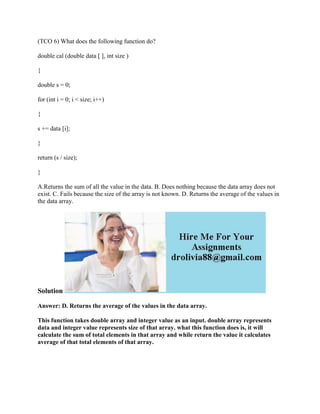Diese Präsentation wurde erfolgreich gemeldet.

# (TCO 6) What does the following function do- double cal (double data -.docx

Anzeige
Anzeige
Anzeige
Anzeige
Anzeige
Anzeige
Anzeige
Anzeige
Anzeige
Anzeige
Anzeige×

1 von 2 Anzeige

# (TCO 6) What does the following function do- double cal (double data -.docx

(TCO 6) What does the following function do?
double cal (double data [ ], int size )
{
double s = 0;
for (int i = 0; i < size; i++)
{
s += data [i];
}
return (s / size);
}
A.Returns the sum of all the value in the data. B. Does nothing because the data array does not exist. C. Fails because the size of the array is not known. D. Returns the average of the values in the data array.
Solution
Answer: D. Returns the average of the values in the data array.
This function takes double array and integer value as an input. double array represents data and integer value represents size of that array. what this function does is, it will calculate the sum of total elements in that array and while return the value it calculates average of that total elements of that array.
.

(TCO 6) What does the following function do?
double cal (double data [ ], int size )
{
double s = 0;
for (int i = 0; i < size; i++)
{
s += data [i];
}
return (s / size);
}
A.Returns the sum of all the value in the data. B. Does nothing because the data array does not exist. C. Fails because the size of the array is not known. D. Returns the average of the values in the data array.
Solution
Answer: D. Returns the average of the values in the data array.
This function takes double array and integer value as an input. double array represents data and integer value represents size of that array. what this function does is, it will calculate the sum of total elements in that array and while return the value it calculates average of that total elements of that array.
.

Anzeige
Anzeige

### (TCO 6) What does the following function do- double cal (double data -.docx

1. 1. (TCO 6) What does the following function do? double cal (double data [ ], int size ) { double s = 0; for (int i = 0; i < size; i++) { s += data [i]; } return (s / size); } A.Returns the sum of all the value in the data. B. Does nothing because the data array does not exist. C. Fails because the size of the array is not known. D. Returns the average of the values in the data array. Solution Answer: D. Returns the average of the values in the data array. This function takes double array and integer value as an input. double array represents data and integer value represents size of that array. what this function does is, it will calculate the sum of total elements in that array and while return the value it calculates average of that total elements of that array.